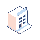• 가격

# Timedelta(months=record.duration)

I want to calculate period in months depends on a fields value

I type that :

`start_date = fields.Datetime('Start Date')end_date = fields.Datetime('End Date', compute='_get_end_date')duration = fields.Integer(string='Duration')`
`@api.depends('start_date', 'duration')def _get_end_date(self):    for record in self:        record.end_date = False        if record.start_date and record.duration:            record.end_date = record.start_date + \                              timedelta(months=record.duration)`

if I type timedelta as hour or days it work
any help?##### 2응답

timedelta doesn't have Months parameter, it only have the below:

Syntax : datetime.timedelta(days=0, seconds=0, microseconds=0, milliseconds=0, minutes=0, hours=0, weeks=0) Returns : Date

So you can use  relativedelta, and the below is the parameters

classdateutil.relativedelta.relativedelta(dt1=Nonedt2=Noneyears=0months=0days=0leapdays=0weeks=0hours=0minutes=0seconds=0microseconds=0year=Nonemonth=Noneday=Noneweekday=Noneyearday=Nonenlyearday=Nonehour=Noneminute=Nonesecond=Nonemicrosecond=None)

```from dateutil.relativedelta import relativedelta

record.end_date = record.start_date + relativedelta(months=record.duration)

```try to use relativedelta instead of timedelta

from dateutil.relativedelta import relativedelta

new_date = old_date + relativedelta(months=5)